Worksheet Solution: Give And Take

# Worksheet Solution: Give And Take - Notes | Study Mathematics for Class 3: NCERT - Class 3

 1 Crore+ students have signed up on EduRev. Have you?

Q.1. Subtraction
(i) 10  -  7  =  _____
Ans.
3

(ii) 28  -   6  =  _____
Ans.
22

(iii) 19  -  9  =  _____
Ans.
10

(iv) 9  -  4  =  _____
Ans.
5

(v) 18  -  5  =  _____
Ans.
13

(vi) 15  -  3  =  _____
Ans.
12

(vii)  20  -  5  =  _____
Ans.
15

(viii)  33  -  3  =  _____
Ans.
30

(ix)  _____  -  7  =  11
Ans.
18

(x)  _____  -  5  = 6
Ans.
11

(xi) _____  -  4  =  8
Ans.
12

(xii) _____  -  6  =  9
Ans.
15

(xiii) _____  -  4  =  14
Ans.
18

(xiv) _____  -  1  =  16
Ans.
17

(xv) 29  -  _____  =  22
Ans.
7

(xvi) 40  -  _____  =  32
Ans.
8

(xvii)  15  -  _____  =  11
Ans.
4

(xviii)  9  -  _____  =  0
Ans.
9

(xix) 28  -  _____  =  18
Ans.
10

(xx)  37  -  _____  =  25
Ans.
12

Q.2. Subtract to find the differences.
(a)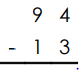Ans.
8   1

(b)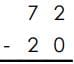Ans.
5   2

(c)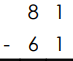Ans.
2   0

(d)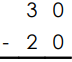Ans.
1   0

(e)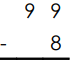Ans.
9   1

(f)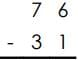Ans.
4   5

(g)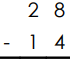Ans.
1   4

(h)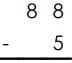Ans.
8   3

(i)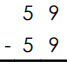Ans.
0

(j)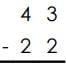Ans.
2  1

(k) Amy has 36 markers. 12 do not work. How many markers do work?
Ans.
24 markers

(l) There are 25 kids in Mr. Ying's class. 13 are girls. How many are boys?
Ans.
12 boys

Q.3.
(i) 31  +  7  =  _____
Ans.
38

(ii) 28  +  3  =  _____
Ans.
31

(iii) 64  +  9  =  _____
Ans.
73

(iv) 56  +  4  =  _____
Ans.
60

(v) 42  +  9  =  _____
Ans.
51

(vi) 15  +  8  =  _____
Ans.
23

(vii)  23  +  5  =  _____
Ans.
28

(viii)  71  +  3  =  _____
Ans.
74

(ix)  40  +  7  =  _____
Ans.
47

(x)  83  +  5  =  _____
Ans.
88

(xi) 96  +  4  =  _____
Ans.
100

(xii) 77  +  6  =  _____
Ans.
83

(xiii) 25  +  9  =  _____
Ans.
34

(xiv) 57  +  3  =  _____
Ans.
60

(xv) 79  +  1  =  _____
Ans.
80

(xvi) 19  +  8  =  _____
Ans.
27

(xvii)  67  +  7  =  _____
Ans.
74

(xviii)  49  +  9  =  _____
Ans.
58

(xix) 90  +  8  =  _____
Ans.
98

(xx)  35  +  2  =  _____
Ans.
37

The document Worksheet Solution: Give And Take - Notes | Study Mathematics for Class 3: NCERT - Class 3 is a part of the Class 3 Course Mathematics for Class 3: NCERT.
All you need of Class 3 at this link: Class 3

## Mathematics for Class 3: NCERT

52 videos|84 docs|44 tests
 Use Code STAYHOME200 and get INR 200 additional OFF

## Mathematics for Class 3: NCERT

52 videos|84 docs|44 tests

Track your progress, build streaks, highlight & save important lessons and more!

,

,

,

,

,

,

,

,

,

,

,

,

,

,

,

,

,

,

,

,

,

;